The relationship between force and position is shown in the figure given (in one dimensional case). The work done by the force in displacing a body from x = 1 cm to x = 5 cm is -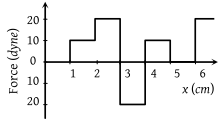(1) 20 ergs

(2) 60 ergs

(3) 70 ergs

(4) 700 ergs

Concept Questions :-

Work done by variable force
High Yielding Test Series + Question Bank - NEET 2020

Difficulty Level:

The pointer reading v/s load graph for a spring balance is as given in the figure. The spring constant is-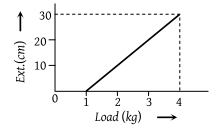(1) 0.1 N/cm

(2) 5 N/cm

(3) 0.3 N/cm

(4) 1 N/cm

Concept Questions :-

Elastic potential energy
High Yielding Test Series + Question Bank - NEET 2020

Difficulty Level:

Adjacent figure shows the force-displacement graph of a moving body, the work done in displacing body from x = 0 to x = 35 m is equal to-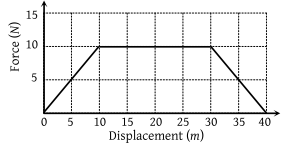(1) 50 J

(2) 25 J

(3) 287.5 J

(4) 200 J

Concept Questions :-

Work done by variable force
High Yielding Test Series + Question Bank - NEET 2020

Difficulty Level:

A 10kg mass moves along x-axis. Its acceleration as a function of its position is shown in the figure. What is the total work done on the mass by the force as the mass moves from x = 0 to x = 8 cm ?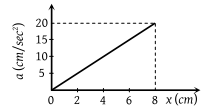(1) 8 × 10–2 joules

(2) 16 × 10–2 joules

(3) 4 × 10–4 joules

(4) 1.6 × 10–3 joules

Concept Questions :-

Work done by variable force
High Yielding Test Series + Question Bank - NEET 2020

Difficulty Level:

A toy car of mass 5 kg moves up a ramp under the influence of force F plotted against displacement x. The maximum height attained is given by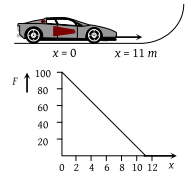(1) ymax = 20 m

(2) ymax = 15 m

(3) ymax = 11 m

(4) ymax = 5 m

Concept Questions :-

Gravitational Potential energy
High Yielding Test Series + Question Bank - NEET 2020

Difficulty Level:

The graph between the resistive force F acting on a body and the distance covered by the body is shown in the figure. The mass of the body is 25 kg and initial velocity is 2 m/s. When the distance covered by the body is 4m, its kinetic energy would be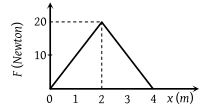(1) 50 J

(2) 40 J

(3) 20 J

(4) 10 J

Concept Questions :-

Work done by variable force
High Yielding Test Series + Question Bank - NEET 2020

Difficulty Level:

A particle of mass 0.1 kg is subjected to a force which varies with distance as shown in fig. If it starts its journey from rest at x = 0 , its velocity at x = 12 m is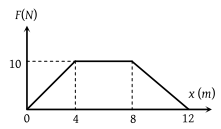(1) 0 m/s

(2) $20\sqrt{2}\text{\hspace{0.17em}}m/s$

(3) $20\sqrt{3}\text{\hspace{0.17em}}m/s$

(4) 40 m/s

Concept Questions :-

Work done by variable force
High Yielding Test Series + Question Bank - NEET 2020

Difficulty Level:

The relation between the displacement X of an object produced by the application of the variable force F is represented by a graph shown in the figure. If the object undergoes a displacement from X = 0.5 m to X = 2.5 m the work done will be approximately equal to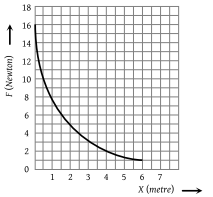(1) 16 J

(2) 32 J

(3) 1.6 J

(4) 8 J

Concept Questions :-

Work done by variable force
High Yielding Test Series + Question Bank - NEET 2020

Difficulty Level:

A particle is given a constant horizontal velocity  from height h. Taking g to be constant every where, kinetic energy E of the particle w. r. t. time t is correctly shown in

(1)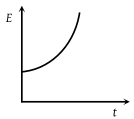(2)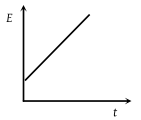(3)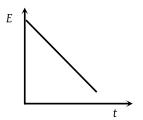(4)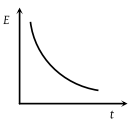Concept Questions :-

Concept of work
High Yielding Test Series + Question Bank - NEET 2020

Difficulty Level:

The adjoining diagram shows the velocity versus time plot for a particle. The work done by the force on the particle is positive from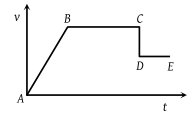(1) A to B

(2) B to C

(3) C to D

(4) D to E

Concept Questions :-

Concept of work